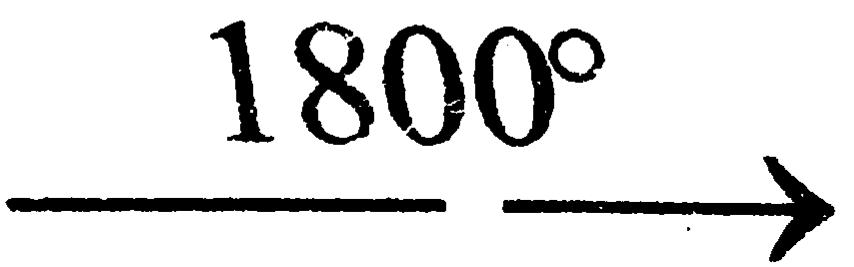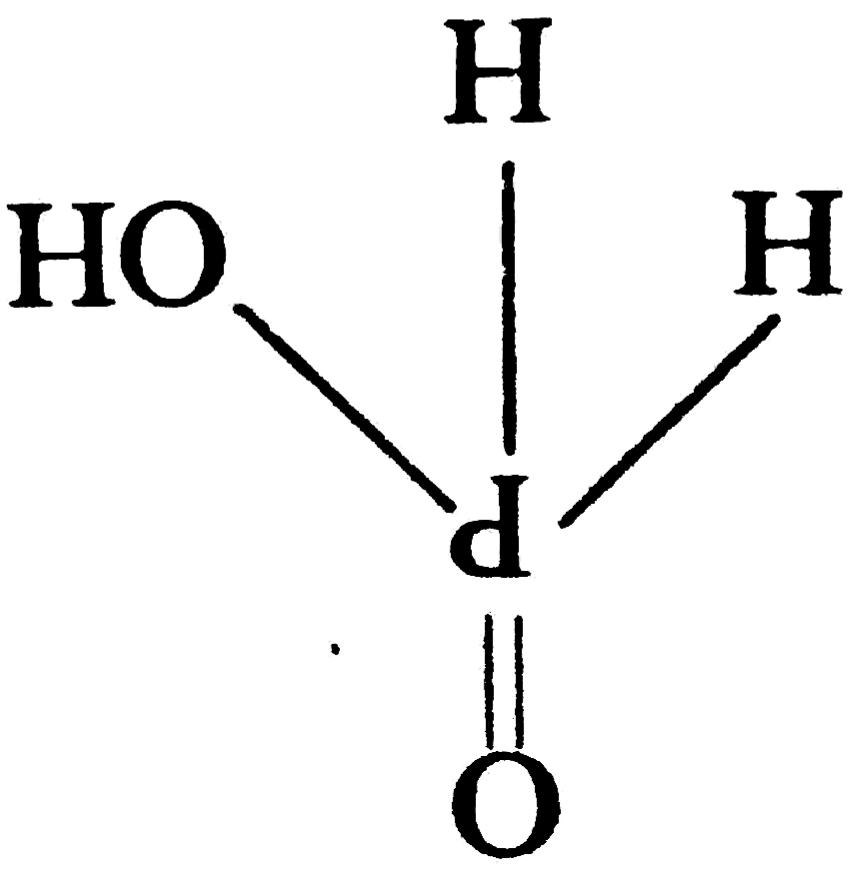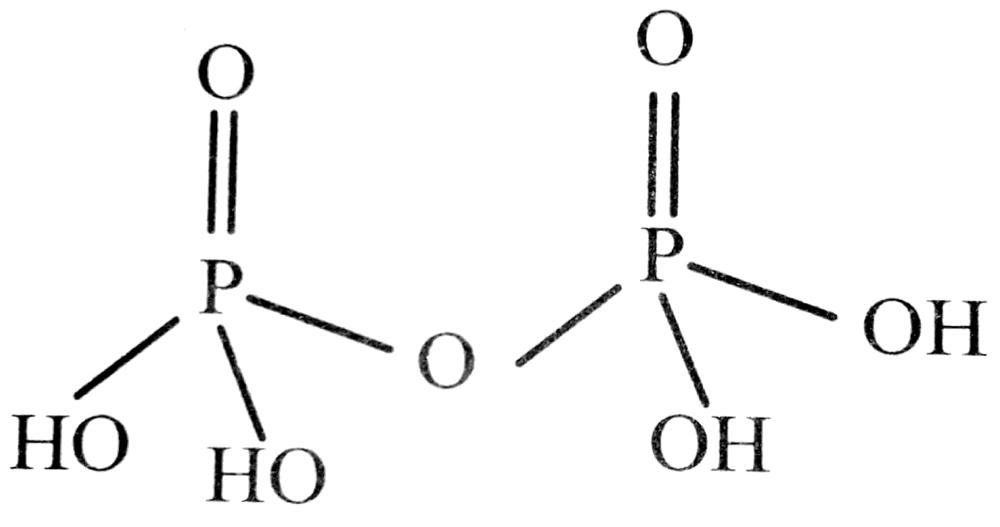7. THE P-BLOCK ELEMENTS

Q. 1. What is laughing gas ? How is it prepared ?

Ans. N2O It is prepared by heating NH4NO3.

Q. 2. Write three uses of Dinitrogen and Ammonia.

Ans. Three uses of Dinitrogen :
(i) For providing as inert atmosphere during many industrial processes where presence of air or O2 is to be avoided.
(ii) For manufacture of HNO3.
(iii) For manufacture of nitrolium.

Three uses of Ammonia :

(i) Used as a retrigeration fluid.
(ii) For remaning grease because NH4OH dissolves grease.
(iii) As laboratory reagent.

Q. 3. White two uses of HNO3 and P4O10

Ans. Two uses of HNO3 :

(i) It is also used for the preparation of nitroglycerin, trinitrotoluene and other organic nitro compounds.
(ii) The majic use of nitric acid is in the manuifacture of ammonium-nitrate for fertilizers.

Two uses of P4O10:

(i) For drying acidic gases.
(ii) For the preparation of SO3 and N2O5.

Q. 4. Write two uses of H2O2 and H2S.

Ans. Two uses of H2O2 :

(i) In bleaching of delicate materials such as silla wolf, cotton, ivory etc.
(ii) As oxidising agent in rocket fuler.

Two uses of H2S:

(i) As a laboratory reagent for the detection of basic redicals in qualitatine analysis.
(ii) As reducing agent.

Q. 5. Explain why NH3 is basic while BiH3 only feebly basic.

Ans. Nitrogen atom has the smallest size and a very high electron density. Therefore, its electron releasing tendency or the basic strength is the maximum. Down the group, there is a gradual increase in atomic size and decrease in the electron density on the central atom. Consequently, the electron releasing tendency or basic strengths of the hydrides decrease in the order as given below.

NH3 > PH3 > AsH3 > Sb H3 > BiH3
Therefore, NH3 is basic while BiH3 is only feebly basic.

Q. 6. How can you separate alumina from silica ? Give equations, if any.

Ans. The powdered alumina is fused with carbon and heated to 1800°C in a current of nitrogen. Aluminium forms its nitride while silica is reduced to silicon which voltilizes off.

Al2O3 + N2 + 3C2AlN + 3CO (g)
SiO2 + 2C → Si↑ + 2CO↑

Q. 7. How is nitrogen prepared in the laboratory ? Write the chemical equations of the reactions involved.

Ans. In the laboratory, nitrogen is prepared by treating an aqueous solution of ammonium chloride with sodium nitrite.

NH4 Cl (aq) + NaNO2 (aq) →
M2 (g) + 2H2O (1) + NaCl (aq)

Q. 8. Nitrogen exists as diatomic molecule but phosphorus as P4. Why ?

Ans. Due to large size, phosphorus has less tendency to form pπ-pπ bonds. So in order to gain stability, it polymerizes and exist as P4 solid while nitrogen complete its octet via pπ-pπ bond formation and N2 is a discrete molecule. In N2, the intermolecular attraction are weak Vander Waal’s forces and hence N2 is a gas.

Q. 9. Boiling point of NH3 is higher than PH3.

Ans. This is because there is intromolecular hydrogen bonding present in NH3 (Due to small size and high electronegativity of N) and not in PH3 (due to large size and less electronegativity of P).

Q. 10. HF is weaker than HI in acetic strength.

Ans. Hydrogen iodide (HI) is more stronger acid then hydrogen floride because iodine (I) is more electronegative than Florine (F) and hence it can donate H+ more easily than Florine (F).

Q. 11. H3PO3 is a di-protic acid.

Ans. Molecular structure of H3PO3 is as following:A diprotic acid is an acid that contains within its molecular structure two hydroxyl group (O – H) from that H3PO3, it is clear that H3PO3 have hydroxyl group is bounded with P. Hence H3PO3 is diprotric acid.

Q. 12. Aniline dissolves in HCl.

Ans. Aniline is somewhat basic. It is not as basic as ammonia, since protonation destroys the interaction between the ring and the lone pair on the nitrogen atom but even still it is sufficiently basic (PKb ~ 9.4) to dissolve in HCl.

Q. 13. PC15 is known but NC15 is not known.

Ans. Nitrogen can’t extend its valence from 3 to 5 due to absence of d-orbital while phosphorous shown penta valences as d-orbital present in its valence cell.

Q. 14. H2O is liquid but H2S is gas.

Ans. In vapour state (~ 100 K). Sulphur partly exists, as S2 molecules like O2 has two unpaired electrons in the antibonding π molecular orbital and exhibits paramagnetism.

Q. 15. Nobel gases are monoatomic in nature.

Ans. Nobel gases are monoatomic in nature. It is because nobel gas make oxidation.

Q. 16. Bleaching of flowers by chlorin is permanent but that by sulphur dioxide temporary.

Ans. In presence of mixture, chlorine acts as an oxidising agent and a bleaching agent.
Cl2 + H2O → HCI → HOCI (unstable)
HOCl → HCI + O (Nascent oxygen)
Coloured matter to → Colourless matter
The bleaching action is permanent due to nascent oxygen. Bleaching action of SO2 in presence of moisture is due to nascent hydrogen.
2SO2 + 2H2O → H2SO4 + 2H
Coloured matter + H → Colour matter So, bleaching action of SO2 is temporary.

Q. 17. Assign an appropriate reason for each of the following statements :
(i) More metal fluorides are ionic in nature than metal chlorides.
(ii) Solid phosphorus pentachloride exhibits some ionic character.

Ans. (i) ‘F’ is more electronegative than ‘Cl’, therefore, fluroides are more ionic metal chlorides.
(ii) PCl5 exhibits some ionic character because it exists as [PCl+4] [PCl6] in solid state.

Q. 18. Why does the reactivity of nitrogen differ from phosphorus ?

Ans. The reactivity of Nitrogen differs from Phosphorus due to its
(i) small size
(ii) high ionisation enthalpy and electronegativity
(iii) non-availability of d-orbitals with N
(iv) Nitrogen has unique ability to from pπ-pπ multiple bonds with itself and with other elements having small size and high electronegativity.

Q. 19. Discuss the trends in chemical reactivity of group 15 elements.

Ans. N differs from other members of the group in ‘its chemical reactivity. Some important trends in the chemical reactivity of group 15 elements are as follow.

Hydrides: All the elements of Group 15 form volatile hydrides of the formula EH3 where E = N, P, As, Sb, Bi. The lighter elements also from hydrides of the formula E2H. N forms HN3.

Oxides : N has a strong tendency to from pπ-pπ multiple bonding with N and O atoms, whereas other elements of this group do not. Actually N form five oxides with oxidation states ranging from + 1 to + 5 (N2O, NO, N2O3, NO2/N2O4, N2O5 respectively) while other elements form oxides only in + 3 and + 5 oxidation states only slike P4O6 and P4O10]

Halides : The elements of Group 15 form halider to two types Trihalides and Pentahalides : EX3 where E = N. P. As, Sb, Bi and X = F, C1, Br and I. EX5 where E = P, As, Sb, Bi

Q. 20. Why are pentahalides more covalent than trihalides ?

Ans. Higher the positive oxidation of central atom, [+5 and +3 in pentahalides and trihalides respectively) more will be its polarising power which, in turn, increases the covalent character of bond formed between the central atom and the other atom.

Q. 21. Why is BiH3 the strongest reducing agent amongst all the hydrides of Group 15 elements ?

Ans. BiH3 is the strongest reducing agent amongst all the hydrides of Group 15, because it is the least stable out of all hydrides of Group 15 elements.

Q. 22. Why is N2 less reactive at room temperature ?

Ans. The bond enthalpy of triple bond in N ≡ N is very high due to pπ-pπ overlap. Hence N2 is less reactive at room temperature. It reacts only at high temperature.

Q. 23. Why is nitrous acid oxidant as well as reductant.

Ans. The oxidation number of N is nitrous acid (H— O-N = O) is + 3 which lies in between its lowest oxidation of – 3 and maximum oxidation state of + 5. Since Ox. no. of + 3 in HNO2 can both be decreased as well as increased. There it acts both as an oxidant and a reductant.

Q. 24. SiFis known but SiClis not. Why is it so ?

Ans. (i) Due to smaller size of F as compard to Cl, steric repulsions will be less in SiFthan in SiCl.
(ii) Bacause of smaller Si-F bond as compared to Si-cl bond the intraction of Flone pair of electrons with Si is stronger than that of Cl ions pairs.

Q. 25. Write the chemical equation to show that chlorine gas can be prepared from bleaching powder.

Ans. Bleaching powder reacts with dilute acids like H2SO4, HCl etc. liberate Cl2 gas.

CaOCl2 + H2SO4 → CaSO4 + H2O + Cl2 (bleaching powder)

Q. 26. Oxygen is diatomic and geseous in nature, explain.

Ans. Due to small size and high electronegativity, oxygen forms pπ – pπ double bond and hence exists as diatomic molecules. Since these diatomic molecules are held together by weak V and der Waals forces of attraction which can be easily broken by collisions of the molecule at room temperature, therfore I2 is a gas.

Q. 27. Give reasons for the least reactivity of nitrogen molecule.

Ans. Due to the presence of triple bond between the two nitrogen atoms (N = N), the bond dissociation energy of N2 is very high. Hence N2 is least reactive.

Q. 28. Why does argon not from diatomic molecules like oxygen and nitrogen ?

Ans. All the orbitals which are occupied by electrons are completely filled in argon and hence it has no tendency to gain, loss, or share electrons with other atoms of Ar to form diatomic molecules. O and , on the other hand, have unpaired electrons and hence share with other O and N atom respectively to form O2 and N2 molecules.

Q. 29. How is pyrophosphoric acid related to orthophosphoric acid ? Write its structure.

Ans. Pyrophosphoric acid (H4P2O7) is the anhydride of orthophosphoric acid and is obtained by removal of a molecule of H2O from two molecules of H3PO4. The structure of pyrophosphoric acid (H4P2O7) isQ. 30. Why is white phosphorus kept under water? Name the three series of salts formed when orthophosphoric acid reacts with sodium hydroxide.

Ans. (i) Ignition temperature of white phosphorus is very low (303 K). Therefore, on exposure to air, it spontaneously catches fire forming P4O10.
(ii) NaH2PO4, Na2HPO4, and Na3PO4.

Q. 31. Noble gases are mostly inert. Assign reasons.

Ans. Noble gases are chemically inert because of the following reasons.
(i) They have completely filled ns2np6 electronic configuration in their valence shell [except He = lS2]
(ii) They have high ionisation enthalpies.
(iii) Electron affinities of noble gases are almost zero.

Q. 32. Why interhalogens are more reactive than halogens ?

Ans. This is because the bond in the interhalogen [A-X] is weaker than X-X bond in the halogens. This is due to less effective overlapping or orbitals of dissimilar atoms than those between similar atoms.

Q.33. Why HF is stored in wax coated glass bottles ?

Ans. This is because HF does not attack wax, but reacts with glass. It dissolves SiO2 present in glass forming hydroflurosilicic acid.

SiO2 + 6 HF → H2SiF6 + 2H2O

## Class 12th Chemistry Short Type Question English

 S.N CHEMISTRY SHORT TYPE QUESTION 2022 1 SOLID STATE 2 SOLUTION 3 ELECTROCHEMISTRY 4 CHEMICAL KINETICS 5 SURFACE CHEMISTRY 6 GENERAL PRINCIPLES AND PROCESSES OF ISOLATION OF ELEMENTS 7 THE P-BLOCK ELEMENTS 8 THE D- AND f-BLOCK ELEMENTS 9 CO-ORDINATION COMPOUNDS 10 HALOALKANES AND HALOARENES 11 ALCOHOLS, PHENOLS AND ETHERS 12 ALDEHYDES, KETONES AND CARBOXYLIC ACIDS 13 AMINES 14 BIOMOLECULES 15 POLYMERS 16 CHEMISTRY IN EVERYDAY LIFE